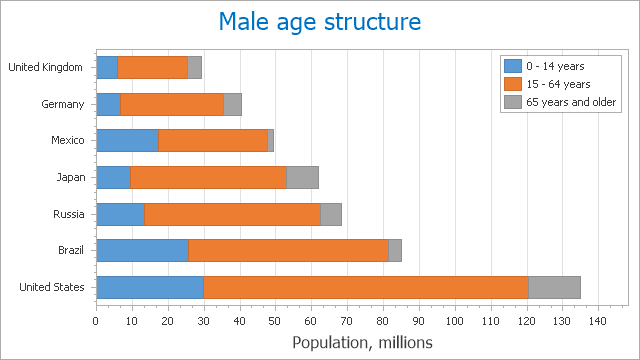# Stacked Bar Chart

## Short Description

A StackedBarSeriesView object is used to display the Stacked Bar Chart. This chart type belongs to the Bar and Column Series View type. This view displays all points of its series. Each bar contains multiple series points (categories) that are stacked above each other, so that the total bar height is the total value of all series points.

A Stacked Bar chart is shown in the image below. Note that this chart type is based upon the XYDiagram, and so it can be rotated to show bars either vertically or horizontally.Note

A Stacked Bar chart can display series that contain data points with positive or negative values. A series with positive values, however, is stacked only with other series that contain positive values; and a series with negative values is stacked with series that contain negative values.

Note that if a series contains data points with both positive and negative values, it is treated as a series with positive values, while all its negative values are treated as zeros.

## Chart Type Characteristics

The table below lists the main characteristics of this chart type.

Feature Value
Series View type StackedBarSeriesView
Diagram type 2D-XYDiagram
Number of arguments per series point 1
Number of values per series point 1

Note

For information on which chart types can be combined with the Stacked Bar Chart, refer to the following help topic: Combining Different Series Views.

## Example

The following example creates a ChartControl with two series of the StackedBarSeriesView type, and adds this chart to a form at runtime. Before proceeding with this example, first create a Windows Forms Application in Visual Studio, and add all required assemblies to the project’s References list.

``````using System;
using System.Windows.Forms;
using DevExpress.XtraCharts;
// ...

private void Form1_Load(object sender, EventArgs e) {
// Create a new chart.
ChartControl stackedBarChart = new ChartControl();

// Create two stacked bar series.
Series series1 = new Series("Series 1", ViewType.StackedBar);
Series series2 = new Series("Series 2", ViewType.StackedBar);

// Add both series to the chart.
stackedBarChart.Series.AddRange(new Series[] { series1, series2 });

// Access the view-type-specific options of the series.
((StackedBarSeriesView)series1.View).BarWidth = 0.8;

// Access the type-specific options of the diagram.
((XYDiagram)stackedBarChart.Diagram).EnableAxisXZooming = true;

// Hide the legend (if necessary).
stackedBarChart.Legend.Visible = false;

// Add a title to the chart (if necessary).